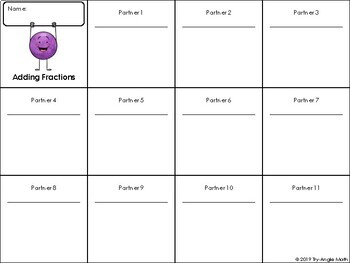# Fraction Operations Poly-Partners ActivitySubject
Resource Type
File Type

PDF

(20 MB|30 pages)
Product Rating
Standards
• Product Description
• StandardsNEW

Subtracting Fractions

Multiplying Fractions

Dividing Fractions

Comparing Fractions

Each student gets a fraction card. I hole punch each one and create a necklace with yarn.

Students pair up, write their fractions, and add, subtract, multiply, divide, or compare!

Students switch partners and repeat.

I created this activity to get students moving and to get students to socialize with students other than their friends. I want all of my students to know how to be kind and get along with ALL students.

This includes:

~ teacher directions

~ student directions for each operation

~ 48 fraction cards - this allows you to differentiate the difficulty within your classroom

~ recording sheets for student work

Since answers will vary, answer keys are not included. I recommend checking 2-4 random problems.

Interpret and compute quotients of fractions, and solve word problems involving division of fractions by fractions, e.g., by using visual fraction models and equations to represent the problem. For example, create a story context for (2/3) ÷ (3/4) and use a visual fraction model to show the quotient; use the relationship between multiplication and division to explain that (2/3) ÷ (3/4) = 8/9 because 3/4 of 8/9 is 2/3. (In general, (𝘢/𝘣) ÷ (𝘤/𝘥) = 𝘢𝘥/𝘣𝘤.) How much chocolate will each person get if 3 people share 1/2 lb of chocolate equally? How many 3/4-cup servings are in 2/3 of a cup of yogurt? How wide is a rectangular strip of land with length 3/4 mi and area 1/2 square mi?
Apply and extend previous understandings of multiplication to multiply a fraction or whole number by a fraction.
Add and subtract fractions with unlike denominators (including mixed numbers) by replacing given fractions with equivalent fractions in such a way as to produce an equivalent sum or difference of fractions with like denominators. For example, 2/3 + 5/4 = 8/12 + 15/12 = 23/12. (In general, 𝘢/𝘣 + 𝘤/𝘥 = (𝘢𝘥 + 𝘣𝘤)/𝘣𝘥.)
Total Pages
30 pages
N/A
Teaching Duration
1 Week
Report this Resource to TpT
Reported resources will be reviewed by our team. Report this resource to let us know if this resource violates TpT’s content guidelines.\$1.00
List Price:
\$2.00
You Save:
\$1.00